JAJSD67A April   2016  – November 2016

PRODUCTION DATA.

1. 特長
2. アプリケーション
3. 概要
4. 改訂履歴
5. Device Comparison Table
6. Pin Configuration and Functions
7. Specifications
8. Parameter Measurement Information
9. Detailed Description
1. 9.1 Overview
2. 9.2 Functional Block Diagrams
3. 9.3 Feature Description
4. 9.4 Device Functional Modes
1. 9.4.1 Device Protection System
10. 10Application and Implementation
1. 10.1 Application Information
2. 10.2 Typical Applications
1. 10.2.1 Stereo BTL Application
2. 10.2.2 Typical Application, Single Ended (1N) SE
3. 10.2.3 Typical Application, Differential (2N), PBTL (Outputs Paralleled before LC filter)
3. 10.3 Typical Application, Differential (2N), PBTL (Outputs Paralleled after LC filter)
11. 11Power Supply Recommendations
1. 11.1 Power Supplies
2. 11.2 Powering Up
3. 11.3 Powering Down
4. 11.4 Thermal Design
12. 12Layout
13. 13デバイスおよびドキュメントのサポート
14. 14メカニカル、パッケージ、および注文情報

#### パッケージ・オプション

デバイスごとのパッケージ図は、PDF版データシートをご参照ください。

• DDW|44

## 7 Specifications

### 7.1 Absolute Maximum Ratings

over operating free-air temperature range (unless otherwise noted) (1)
MIN MAX UNIT
Supply voltage BST_X to GVDD_X(2) –0.3 43 V
VDD to GND –0.3 13.2 V
GVDD_X to GND(2) –0.3 13.2 V
PVDD_X to GND(2) –0.3 43 V
DVDD to GND –0.3 4.2 V
AVDD to GND –0.3 8.5 V
VBG to GND -0.3 4.2 V
Interface pins OUT_X to GND(2) –0.3 43 V
BST_X to GND(2) –0.3 55.5 V
OC_ADJ, M1, M2, OSC_IOP, OSC_IOM, FREQ_ADJ, C_START, to GND –0.3 4.2 V
RESET, FAULT, CLIP_OTW, CLIP to GND –0.3 4.2 V
INPUT_X to GND –0.3 7 V
Continuous sink current, RESET, FAULT, CLIP_OTW, CLIP, RESET to GND 9 mA
TJ Operating junction temperature range 0 150 °C
Tstg Storage temperature range –40 150 °C
Stresses beyond those listed under Absolute Maximum Ratings may cause permanent damage to the device. These are stress ratings only, which do not imply functional operation of the device at these or any other conditions beyond those indicated under Recommended Operating Conditions. Exposure to absolute-maximum-rated conditions for extended periods may affect device reliability.
These voltages represents the DC voltage + peak AC waveform measured at the terminal of the device in all conditions.

### 7.2 ESD Ratings

VALUE UNIT
VESD Electrostatic discharge Human body model (HBM), per ANSI/ESDA/JEDEC JS-001, all pins (1) ±1000 V
Charged device model (CDM), per JEDEC specification JESD22-C101, all pins(2) ±250 V
JEDEC document JEP155 states that 500-V HBM allows safe manufacturing with a standard ESD control process.
JEDEC document JEP157 states that 250-V CDM allows safe manufacturing with a standard ESD control process.

### 7.3 Recommended Operating Conditions

over operating free-air temperature range (unless otherwise noted)
MIN TYP MAX UNIT
PVDD_x Half-bridge supply DC supply voltage 12 30 31.5 V
GVDD_x Supply for logic regulators and gate-drive circuitry DC supply voltage 10.8 12 13.2 V
VDD Digital regulator supply voltage DC supply voltage 10.8 12 13.2 V
RL(BTL) Load impedance Output filter inductance within recommended value range 2.7 4 Ω
RL(SE) 1.5 3
RL(PBTL) 1.6 2
LOUT(BTL) Output filter inductance Minimum output inductance at IOC 5 μH
LOUT(SE) 5
LOUT(PBTL) 5
FPWM PWM frame rate selectable for AM interference avoidance; 1% Resistor tolerance Nominal 430 450 470 kHz
AM1 475 500 525
AM2 575 600 625
R(FREQ_ADJ) PWM frame rate programming resistor Nominal; Master mode 29.7 30 30.3
AM1; Master mode 19.8 20 20.2
AM2; Master mode 9.9 10 10.1
CPVDD PVDD close decoupling capacitors 1.0 μF
ROC Over-current programming resistor Resistor tolerance = 5% 22 30
ROC(LATCHED) Over-current programming resistor Resistor tolerance = 5% 47 64
V(FREQ_ADJ) Voltage on FREQ_ADJ pin for slave mode operation Slave mode 3.3 V
TJ Junction temperature 0 125 °C

### 7.4 Thermal Information

THERMAL METRIC(1) TPA3244 UNIT
DDV 44-PINS HTSSOP
JEDEC STANDARD 4 LAYER PCB
RθJA Junction-to-ambient thermal resistance 23.0 °C/W
RθJC(top) Junction-to-case (top) thermal resistance 9.1 °C/W
RθJB Junction-to-board thermal resistance 3.9 °C/W
ψJT Junction-to-top characterization parameter 0.1 °C/W
ψJB Junction-to-board characterization parameter 3.9 °C/W
RθJC(bot) Junction-to-case (bottom) thermal resistance 0.3 °C/W

### 7.5 Electrical Characteristics

PVDD_X = 30 V, GVDD_X = 12 V, VDD = 12 V, TA (Ambient temperature) = 25°C, fS = 450 kHz, unless otherwise specified.
PARAMETER TEST CONDITIONS MIN TYP MAX UNIT
INTERNAL VOLTAGE REGULATOR AND CURRENT CONSUMPTION
DVDD Voltage regulator, only used as reference node VDD = 12 V 3 3.3 3.6 V
AVDD Voltage regulator, only used as reference node VDD = 12 V 7.8 V
IVDD VDD supply current Operating, 50% duty cycle 40 mA
Idle, reset mode 13
IGVDD_X Gate-supply current per full-bridge 50% duty cycle 15 mA
Reset mode 2
IPVDD_X PVDD idle current per full bridge 50% duty cycle with 10µH Output Filter Inductors 12.5 mA
Reset mode, No switching 1 mA
RIN Input resistance 24
VIN Maximum input voltage swing 7 V
IIN Maximum input current 1 mA
G Inverting voltage Gain VOUT/VIN 20 dB
OSCILLATOR
fOSC(IO+) Nominal, Master Mode FPWM × 6 2.58 2.7 2.82 MHz
AM1, Master Mode 2.85 3 3.15
AM2, Master Mode 3.45 3.6 3.75
VIH High level input voltage 1.86 V
VIL Low level input voltage 1.45 V
OUTPUT-STAGE MOSFETs
RDS(on) Drain-to-source resistance, low side (LS) TJ = 25°C, Includes metallization resistance,
GVDD = 12 V
65
Drain-to-source resistance, high side (HS) 65
I/O PROTECTION
Vuvp,VDD,GVDD Undervoltage protection limit, GVDD_x and VDD 9.5 V
Vuvp,VDD, GVDD,hyst (1) 0.6 V
Vuvp,PVDD Undervoltage protection limit, PVDD_x 10 V
Vuvp,PVDD,hyst (1) 0.6 V
OTW Overtemperature warning, CLIP_OTW(1) 115 125 135 °C
OTWhyst (1) Temperature drop needed below OTW temperature for CLIP_OTW to be inactive after OTW event. 25 °C
OTE(1) Overtemperature error 145 155 165 °C
OTEhyst (1) A reset needs to occur for FAULT to be released following an OTE event 25 °C
OTE-OTW(differential) (1) OTE-OTW differential 30 °C
OLPC Overload protection counter fPWM = 450 kHz 2.3 ms
IOC Overcurrent limit protection Resistor – programmable, nominal peak current in 1Ω load, ROCP = 22 kΩ 14 A
IOC(LATCHED) Overcurrent limit protection Resistor – programmable, peak current in 1Ω load, ROCP = 47kΩ 14 A
IDCspkr DC Speaker Protection Current Threshold BTL current imbalance threshold 1.5 A
IOCT Overcurrent response time Time from switching transition to flip-state induced by overcurrent. 150 ns
IPD Output pulldown current of each half Connected when RESET is active to provide bootstrap charge. Not used in SE mode. 3 mA
STATIC DIGITAL SPECIFICATIONS
VIH High level input voltage M1, M2, OSC_IOP, OSC_IOM, RESET 1.9 V
VIL Low level input voltage 0.8 V
Ilkg Input leakage current 100 μA
OTW/SHUTDOWN (FAULT)
RINT_PU Internal pullup resistance, CLIP_OTW to DVDD, FAULT to DVDD 20 26 32
VOH High level output voltage Internal pullup resistor 3 3.3 3.6 V
VOL Low level output voltage IO = 4 mA 200 500 mV
Device fanout CLIP_OTW, FAULT No external pullup 30 devices
Specified by design.

### 7.6 Audio Characteristics (BTL)

PCB and system configuration are in accordance with recommended guidelines. Audio frequency = 1 kHz, PVDD_X = 30 V, GVDD_X = 12 V, RL = 8 Ω, fS = 450 kHz, ROC = 22 kΩ, TA = 25°C, Output Filter: LDEM = 10 μH, CDEM = 1 µF, mode = 00, AES17 + AUX-0025 measurement filters,unless otherwise noted.
PARAMETER TEST CONDITIONS MIN TYP MAX UNIT
PO Power output per channel RL = 8 Ω, 10% THD+N 60 W
RL = 4 Ω, 10% THD+N, Single Channel, 20 seconds duration(1) 110
RL = 8 Ω, 1% THD+N 50
RL = 4 Ω, 1% THD+N, 3 seconds Peak Power(1) 90
RL = 4 Ω, 1% THD+N, Single Channel, 40 seconds Peak Power(1) 90
THD+N Total harmonic distortion + noise 1 W 0.005%
Vn Output integrated noise A-weighted, AES17 filter, Input Capacitor Grounded 60 μV
|VOS| Output offset voltage Inputs AC coupled to GND 20 60 mV
SNR Signal-to-noise ratio(2) 111 dB
DNR Dynamic range 111 dB
Pidle Power dissipation due to Idle losses (IPVDD_X) PO = 0, 4 channels switching(3) 0.38 W
Peak Power rating using TPA3244 EVM
SNR is calculated relative to 1% THD+N output level.
Actual system idle losses also are affected by core losses of output inductors.

### 7.7 Audio Characteristics (SE)

PCB and system configuration are in accordance with recommended guidelines. Audio frequency = 1 kHz, PVDD_X = 30 V, GVDD_X = 12 V, RL = 4 Ω, fS = 450 kHz, ROC = 22 kΩ, TA = 25°C, Output Filter: LDEM = 15 μH, CDEM = 1 µF, MODE = 11, AES17 + AUX-0025 measurement filters, unless otherwise noted.
PARAMETER TEST CONDITIONS MIN TYP MAX UNIT
PO Power output per channel RL = 4 Ω, 10% THD+N 30 W
RL = 3 Ω, 10% THD+N 39
RL = 4 Ω, 1% THD+N 25
RL = 3 Ω, 1% THD+N 32
THD+N Total harmonic distortion + noise 1 W 0.01%
Vn Output integrated noise A-weighted, AES17 filter, Input Capacitor Grounded 100 μV
SNR Signal to noise ratio(1) A-weighted 100 dB
DNR Dynamic range A-weighted 101 dB
Pidle Power dissipation due to idle losses (IPVDD_X) PO = 0, 4 channels switching(2) 0.38 W
SNR is calculated relative to 1% THD+N output level.
Actual system idle losses are affected by core losses of output inductors.

### 7.8 Audio Characteristics (PBTL)

PCB and system configuration are in accordance with recommended guidelines. Audio frequency = 1 kHz, PVDD_X = 30 V, GVDD_X = 12 V, RL = 4 Ω, fS = 450 kHz, ROC = 22 kΩ, TA = 25°C, Output Filter: LDEM = 10 μH, CDEM = 1 µF, MODE = 10, outputs paralleled before LC filter, AES17 + AUX-0025 measurement filters, unless otherwise noted.
PARAMETER TEST CONDITIONS MIN TYP MAX UNIT
PO Power output per channel RL = 4 Ω, 10% THD+N 125 W
RL = 3 Ω, 10% THD+N 160
RL = 4 Ω, 1% THD+N 100
RL = 3 Ω, 1% THD+N 130
THD+N Total harmonic distortion + noise 1 W 0.005%
Vn Output integrated noise A-weighted, AES17 filter, Input Capacitor Grounded 55 μV
SNR Signal to noise ratio(1) A-weighted 112 dB
DNR Dynamic range A-weighted 112 dB
Pidle Power dissipation due to idle losses (IPVDD_X) PO = 0, 4 channels switching(2) 0.38 W
SNR is calculated relative to 1% THD+N output level.
Actual system idle losses are affected by core losses of output inductors.

### 7.9 Typical Characteristics

#### 7.9.1 BTL Configuration

All Measurements taken at audio frequency = 1 kHz, PVDD_X = 30 V, GVDD_X = 12 V, RL = 8 Ω, fS = 450 kHz, ROC = 22 kΩ, TA = 25°C, Output Filter: LDEM = 10 μH, CDEM = 1 µF, mode = 00, AES17 + AUX-0025 measurement filters,unless otherwise noted.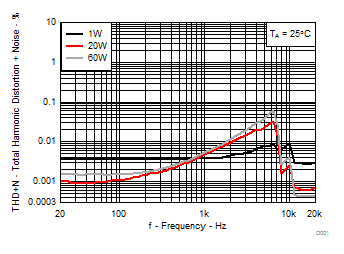RL = 4 Ω P = 1W, 20W, 60W TA = 25°C
Figure 1. Total Harmonic Distortion+Noise vs Frequency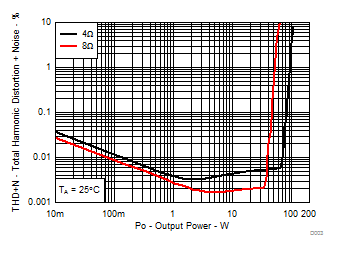RL =4 Ω, 8 Ω TA = 25°C
Figure 3. Total Harmonic Distortion + Noise vs Output Power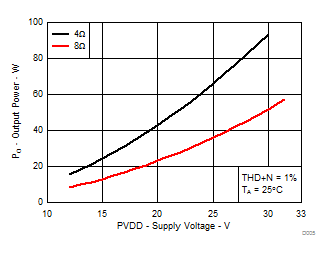RL = 4 Ω, 8 Ω THD+N = 1% TA = 25°C
Figure 5. Output Power vs Supply Voltage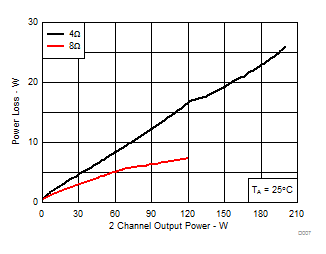RL = 4 Ω, 8 Ω TA = 25°C
Figure 7. System Power Loss vs Output Power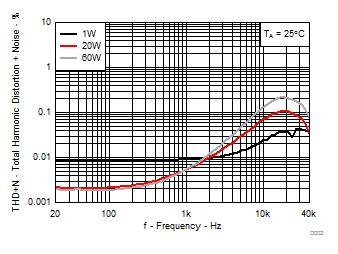RL = 4 Ω P = 1W, 20W, 60W TA = 25°C AUX-0025 filter, 80 kHz analyzer BW
Figure 2. Total Harmonic Distortion+Noise vs Frequency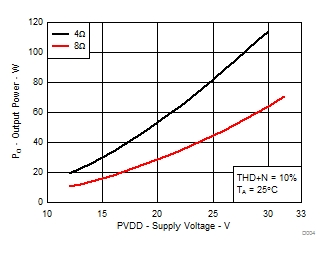RL = 4 Ω, 8 Ω THD+N = 10% TA = 25°C
Figure 4. Output Power vs Supply Voltage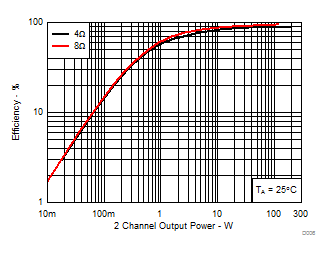RL = 4 Ω, 8 Ω TA = 25°C
Figure 6. System Efficiency vs Output Power8 Ω, VREF = 25.46 V (1% Output power) FFT = 16384 AUX-0025 filter, 80 kHz analyzer BW TA = 25°C
Figure 8. Noise Amplitude vs Frequency

#### 7.9.2 SE Configuration

All Measurements taken at audio frequency = 1 kHz, PVDD_X = 30 V, GVDD_X = 12 V, RL = 4 Ω, fS = 450 kHz, ROC = 22 kΩ, TA = 25°C, Output Filter: LDEM = 15 μH, CDEM = 680 nF, MODE = 11, AES17 + AUX-0025 measurement filters, unless otherwise noted.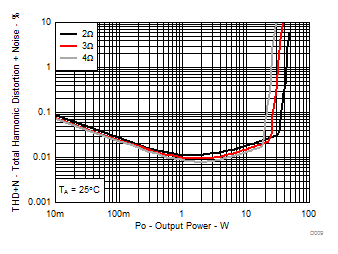RL = 2 Ω, 3Ω, 4Ω TA = 25°C
Figure 9. Total Harmonic Distortion+Noise vs Output PowerRL = 4Ω P = 1W, 5W, 20W TA = 25°C AUX-0025 filter, 80 kHz analyzer BW
Figure 11. Total Harmonic Distortion+Noise vs Frequency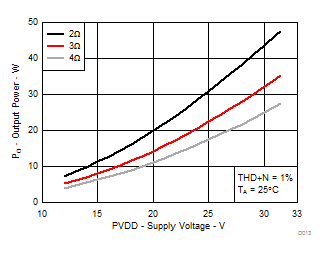RL = 2 Ω, 3Ω, 4Ω THD+N = 1% TA = 25°C
Figure 13. Output Power vs Supply Voltage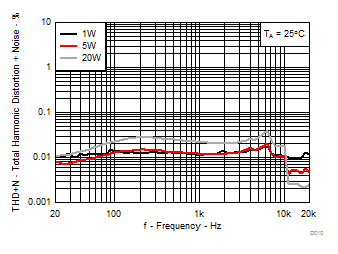RL = 4Ω P = 1W, 5W, 20W TA = 25°C
Figure 10. Total Harmonic Distortion+Noise vs Frequency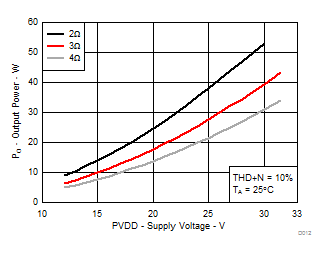RL = 2 Ω, 3Ω, 4Ω THD+N = 10% TA = 25°C
Figure 12. Output Power vs Supply Voltage

#### 7.9.3 PBTL Configuration

All Measurements taken at audio frequency = 1kHz, PVDD_X = 30 V, GVDD_X = 12 V, RL = 4Ω, fS = 450 kHz, ROC = 22 kΩ, TA = 25°C, Output Filter: LDEM = 10 μH, CDEM = 1 µF, MODE = 10, outputs paralleled before LC filter, AES17 + AUX-0025 measurement filters, unless otherwise noted.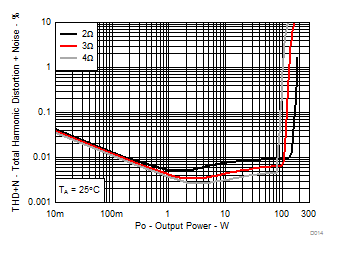RL = 2 Ω, 3Ω, 8Ω TA = 25°C
Figure 14. Total Harmonic Distortion+Noise vs Output Power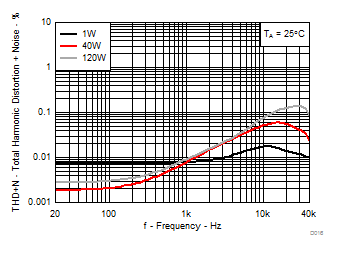RL = 2Ω P = 1W, 40W, 120W TA = 25°C AUX-0025 filter, 80 kHz analyzer BW
Figure 16. Total Harmonic Distortion+Noise vs Frequency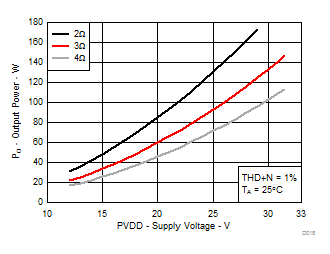RL = 2Ω, 3Ω, 4Ω THD+N = 1% TA = 25°C
Figure 18. Output Power vs Supply Voltage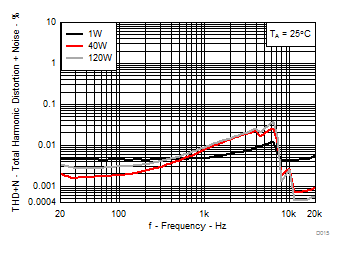RL = 2Ω P = 1W, 40W, 120W TA = 25°C
Figure 15. Total Harmonic Distortion+Noise vs Frequency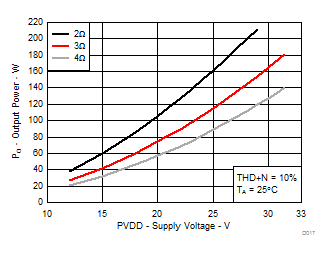RL = 2Ω, 3Ω, 4Ω THD+N = 10% TA = 25°C
Figure 17. Output Power vs Supply Voltage